# Chapter 8 Lines and Angles RD Sharma Solutions Exercise 8.4 Class 9 Maths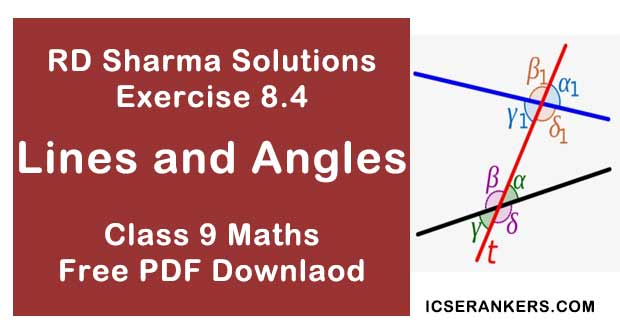Chapter Name RD Sharma Chapter 8 Lines and Angles Exercise 8.4 Book Name RD Sharma Mathematics for Class 10 Other Exercises Exercise 8.1Exercise 8.2Exercise 8.3 Related Study NCERT Solutions for Class 10 Maths

### Exercise 8.4 Solutions

1. In below fig, AB, CD and ∠1 and ∠2 are in the ratio 3 : 2. Determine all angles from 1 to 8.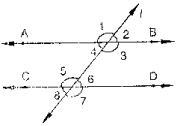Solution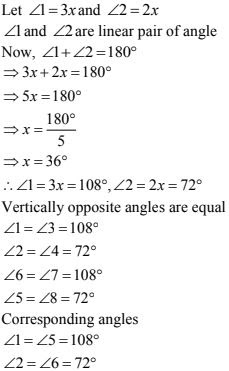2. In the below fig, l, m and n are parallel lines intersected by transversal P at X, Y and Z respectively. Find ∠1, ∠2 and ∠3.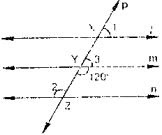Solution
From the given figure:3. In the below fig, AB||CD||EF and GH|| KL. Find ∠HKLSolution
Produce LK to meet GF at N.
Now, alternative angles are equal4. In the below fig, show that AB||EF.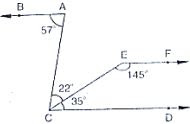Solution
Produce EF to intersect AC at K.
Now, ∠DCE + ∠CEF = 35 + 145 = 180
∴ EF || CD  [∵ Sum of Co - interior angles is 180°] ...(1)
Now, ∠BAC = ∠ACD = 57°
⇒BA || CD  [∵ Alternative angles are equal]
From (1) and (2)
AB || EF  [Lines parallel to the same line are parallel to each other]
Hence, proved.

5. If below fig, if AB || CD and CD || EF, find ∠ACE.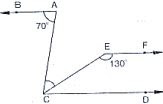Solution
Since EF || CD
∴ ∠EFC + ∠ECD = 180°  [co- interior angles are supplementary]
⇒ ∠ECD = 180° - 130° = 50°
Also BA || CD
⇒ ∠BAC = ∠ACD = 70°  [alternative angles]
But
∠ACE + ∠ECD = 70°
⇒ ∠ACE = 70° - 50° = 20°

6. In the below fig, PQ||AB are PR ||BC. If ∠QPR = 102° , ∠ABC. Give reasons.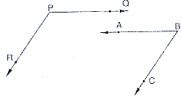Solution
AB is produce to meet PR at K
Since PQ || AB
∠QPR = ∠BKR = 102°  [corresponding angles]
Since PR || BC
∴ ∠RKB + ∠CBK = 180°  [∵ corresponding angles are supplementary]
⇒ ∠CKB = 108 - 102 = 78°
∴ ∠CKB = 78°

7. In the below fig, state which lines are parallel and why ?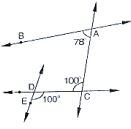Solution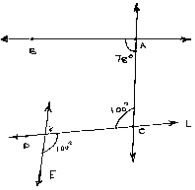Vertically opposite angles are equal
∠EOC = ∠DOK = 100°
Angle ∠DOK = ∠ACO = 100°
Here two lines EK and CA cut by a third line 'l' and the corresponding angles to it are equal
∴ EK || AC.

8. In the below fig, if  l || m, n || p and ∠1 = 85° , find ∠2.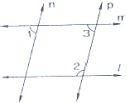Solution
Corresponding angles are equal
∠1 = ∠3 = 85°
By using the property of co-interior angles are supplementary
∠2 + ∠3 = 180°
⇒ ∠2 + 55° = 180°
⇒ ∠2 = 95°
∴ ∠2 = 95°

9. If two straight lines are perpendicular to the same line, prove that they are parallel to each other.
Solution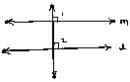Given m perpendicular t and l ⊥ t
∠1 = ∠2 = 90
and m are two lines and it is transversal and the corresponding angles are equal
l || m
Hence, proved

10. Prove that if the to arms of an angle are perpendicular to the two arms of another angle, then the angles are either equal or supplementary.
Solution
Consider be angles AOB and ACB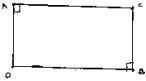Given OA ⊥ AO, OB ⊥ BO
To prove : ∠AOB = ∠ACB (or)
∠AOB + ∠ACB = 180°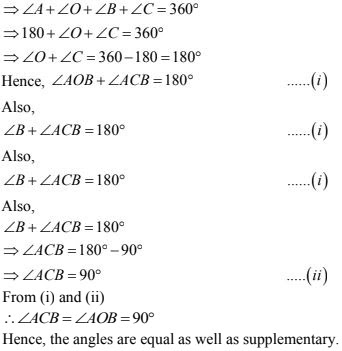11. In the below fig, lines AB and CD are parallel and P is any point as shown in the figure. Show that ∠ABP + ∠CDP = ∠DPB.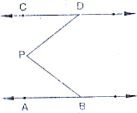Solution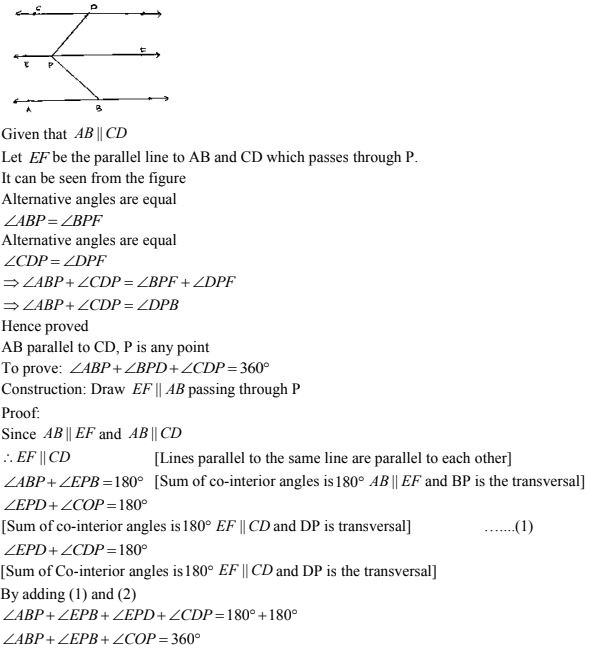12. In the below fig, AB || CD and P is any point shown in the figure. Prove that :
∠ABP + ∠BPD + ∠CDP = 360°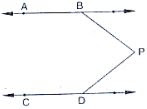Solution
Through P, draw a line PM parallel to AB or CD.
Now,
AB || PM
⇒ ∠ABP + ∠BPM = 180°
And
CD || PM
⇒ ∠MPD + ∠CDP = 180°
Adding (i) and (ii), we get
∠ABP + (∠BPM + ∠MPD) + ∠CDP = 360°
⇒∠ABP + ∠BPD + ∠CDP = 360°

13. Two unequal angles of a parallelogram are in the ratio 2 : 3. Find all its angles in degrees.
Solution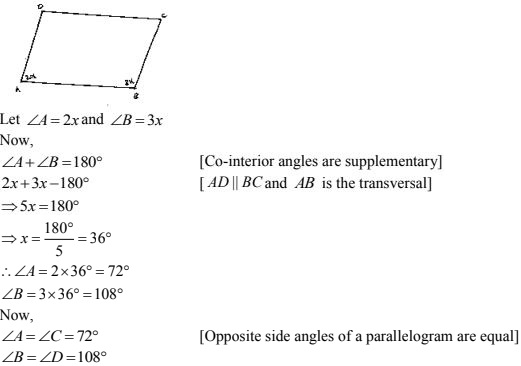14. If each of the two lines is perpendicular to the same line, what kind of lines are they to each other ?
Solution15. In the below fig, ∠1 = 60° and ∠2 = (2/3)rd of a right angle. Prove that l || m.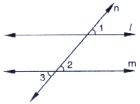Solution°
Given
∠1 = 60°, ∠2 = (2/3)rd to right angle
To prove : parallel to m
Proof ∠1 = 60°
∠2 = 2/3 ×90° = 60°
Since, ∠1 = ∠2 = 60°
∴ Parallel to m as pair of corresponding angles are equal

16. In the below fig, if l || m || n and ∠1 = 60° , find ∠2.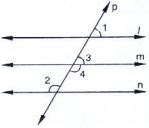Solution
Since  parallel to m and p is the transversal
∴ Given: l || m || n, ∠1 = 60°
To find ∠2
∠1 = ∠3 = 60° [Corresponding angles]
Now,
∠3 and ∠4 are linear pair of angles
∠3 + ∠4 = 180°
⇒ 60° + ∠4 = 180°
⇒ ∠4 = 180° - 60°
⇒ ∠4 = 120°
Also, m || n and P is the transversal
∴ ∠4 = ∠2 = 120° [Alternative interior angle]
Hence ∠2 = 120°

17. Prove that the straight lines perpendicular to the same straight line are parallel to one another.
Solution
Let AB and CD perpendicular to the line MN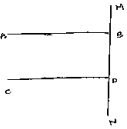∠ABD = 90° [ ∵ AB ⊥ MN] ...(i)
∠CON  = 90° [∵ CD ⊥ MN]  ...(ii)
Now,
∠ABD = ∠CDN = 90°  [From (i) and (ii)]
∴ AB || CD, Since corresponding angles are equal.

18. The opposite sides of a quadrilateral are parallel. If one angle of the quadrilateral is 60°, find the other angles.
Solution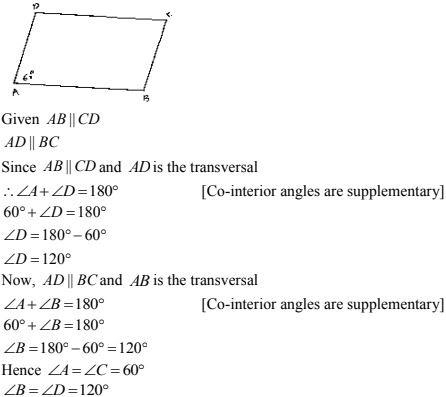19. Two lines AB and CD intersect at O. If ∠AOC + ∠COB + ∠BOD = 270° , find the measures of ∠AOC, ∠COB, ∠BOD and ∠DOA.
Solution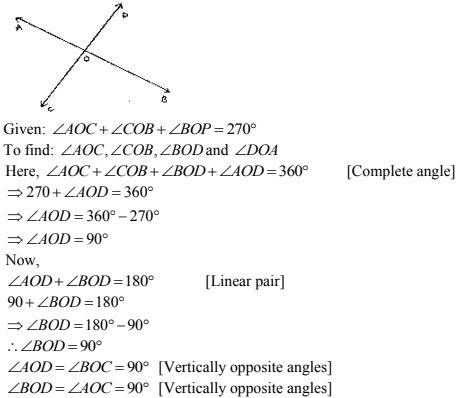20. In the below fig, p is a transversal to lines m and n, ∠2 = 120° and ∠5 = 60° . Prove that m || n.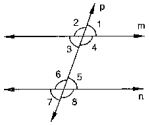Solution
Given that
∠2 = 120°, ∠5 = 60°
To prove
∠2 + ∠1 = 180°  [Linear pair]
⇒ 120° + ∠1= 180°
⇒ ∠1 = 180° - 120°
⇒ ∠1 = 60°
Since ∠1 = ∠5 = 60°
∴ m || n  [As pair of corresponding angles are equal]

21. In the below fig, transversal  intersects two lines m and n, ∠4 = 110° and ∠7 = 65 . Is m || n  ?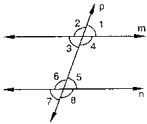Solution
Given,
∠4 = 110° , ∠7 = 65°
To find : Is m || n
Here, ∠7 =∠5 = 65°  [Vertically opposite angle]
Now,
∠4 + ∠5 = 110 + 65 = 175°
∴ m is not parallel  to n as the pair of co - interior angles is not supplementary.

22. Which pair of lines in the below fig, is parallel ? Given reasons.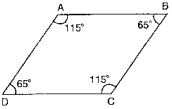Solution
∠A + ∠B = 115 + 65 = 180°
∴ AB || BC  [As sum of co-interior angles we supplementary]
∠B + ∠C  = 65° + 115° = 180°
∴ AB || CD  [As sum of interior angles are supplementary]

23. If l, m, n are three lines such that l || m and n ⊥ l , prove that n ⊥ m.
Solution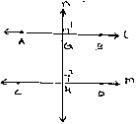Given l || m, n perpendicular
To prove : n ⊥ m
Since  l || m and n intersects them at G and H respectively
∴ ∠1 = ∠2  [Corresponding angles]
But, U = 90° [n ⊥ l]
⇒ ∠2 = 90°
Hence, n perpendicular m

24. In the below fig, arms BA and BC of ∠ABC are respectively parallel to arms ED and EF of ∠DEF. Prove that ∠ABC = ∠DEF.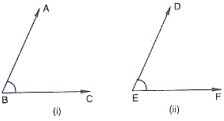Solution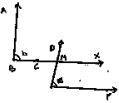Given AB || DE and BC || EF
To prove : ∠ABC = ∠DEF
Construction : Produce BC to x such that it intersect DE at M.
Proof :  Since AB || DE and BX is the transversal
∴ ∠ABC = ∠DMX  [Corresponding angle] ...(i)
Also,
BX || EF and DE is the transversal
∴ ∠DMX = ∠DEF  [Corresponding angles]
From (i) and (ii)
∴ ∠ABC = ∠DEF

25. In the below fig, arms BA and BC of ∠ABC are respectively parallel to arms ED and EF of ∠DEF. Prove that ∠ABC + ∠DEF = 180° .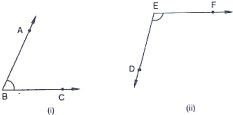SolutionGiven AB || DE, BC || EF
To prove : ∠ABC + ∠DEF = 180°
Construction : produce BC to intersect DE at M
Proof : Since AB || EM and BL is the transversal
∠ABC = ∠EML  [Corresponding angle]
Also,
EF ||  ML and EM is the transversal
By the property of co-interior angles are supplementary
∠DEF + ∠EML  = 180°
From (i) and (ii) we have
∴ ∠DEF + ∠ABC = 180°

26. Which of the following statements are true (T) and which are false (F) ? Give reasons.
(i) If two lines are intersected by a transversal, then corresponding angles are equal.
(ii) If two parallel lines are intersected by a transversal, then alternate interior angles are equal.
(iii) Two lines perpendicular to the same line are perpendicular to each other.
(iv) Two lines parallel to the same line are parallel to each other.
(v) If two parallel lines are intersected by a transversal, then the interior angles on the same side of the transversal are equal.
Solution
(i) False
(ii) True
(iii) False
(iv) True

27. Fill in the blanks in each of the following to make the statement true:
(i) If two parallel lines are intersected by a transversal, then each pair of corresponding angles are ________
(ii) If two parallel lines are intersected by a transversal, then interior angles on the same side of te transversal are _______
(iii) Two lines perpendicular to the same line are _________ to each other.
(iv) Two lines parallel to the same line are _______ to each other.
(v) If a transversal intersects a pair of lines in such a way that a pair of alternate angles are equal, then the liens are _______
(vi) If a transversal intersects a pair of lines in such a way that the sum of interior angles on the same side of transversal is 180°, then the lines are  ______ .
Solution
(i) Equal
(ii) Supplementary
(iii) Parallel
(iv) Parallel
(v) Parallel
(vi) Parallel